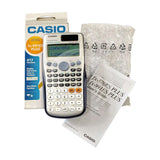# CASIO Scientific Calculator FX-991ES Plus

2 sold in last 8 hours
CP-SS1-648
Rs.4,613.00
Rs.4,613.00

CASIO scientific calculator (FX-991ES Plus), comes with slide-on hard case

The Natural Display (Natural-V.P.A.M.) shows mathematical expressions like roots and fractions as they appear in your textbook, and this increases comprehension because results are easier to understand.

#### Description:

• Power Source: Solar, Battery
• Number of Batteries: 1 LR44 batteries required. (included)
• Item Dimensions L x W x H : 6.38 x 3.03 x 0.43 inches
• Display Type: LCD

#### Standard Functions:

• Fraction calculations
• Combination and permutation
• Statistics (List-based STAT data editor, standard deviation, regression analysis)
• 9 variables
• Table function
• Equation calculations
• Integration/differential calculations
• Matrix calculations
• Vector calculations
• Complex number calculations
• CALC function
• SOLVE function
• Base- n calculation
• Comes with new slide-on hard case

#### New Functions:

• New equation mode
• Random integers

## Customer Reviews

Based on 1 reviewCASIO Scientific Calculator FX-991ES Plus
You have successfully subscribed!
This email has been registered
Top